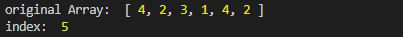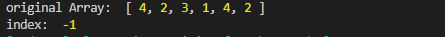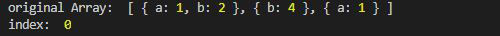# Lodash _.findLastIndex() Function

• Last Updated : 16 Jul, 2020

Lodash is a JavaScript library that works on the top of underscore.js. Lodash helps in working with arrays, strings, objects, numbers, etc.
The _.findLastIndex() function is used to find the element from the right of the array. Therefore giving the index of the last occurrence of the element in the array.

Syntax:

```findLastIndex(array, [predicate=_.identity], fromIndex);
```

Parameter:

• array: It is the original array.
• predicate: It is the function that iterates over each element.
• fromIndex: It is the index after which the searching starts. If from Index is not given then by default it is n-1 where n is the length of the array.

Return Value: It returns the index of the element if found else -1 is returned.

Note: Install lodash module by using command `npm install lodash` before using the code given below.

Example 1:

 `// Requiring the lodash library``const _ = require(``"lodash"``);`` ` `// Original array``let array1 = [4, 2, 3, 1, 4, 2]`` ` `// Using lodash.findLastIndex``let index = _.findLastIndex(array1, (e) => {``    ``return` `e == 2;``});`` ` `// Original Array``console.log(``"original Array: "``, array1)`` ` `// Printing the index``console.log(``"index: "``, index)`

Output:Example 2: When an element is present in the array but the output is -1 because it is present after the from the index. Here fromIndex is 2.

 `// Requiring the lodash library``const _ = require(``"lodash"``);`` ` `// Original array``let array1 = [4, 2, 3, 1, 4, 2]`` ` `// Using lodash.findLastIndex``let index = _.findLastIndex(array1, (e) => {``    ``return` `e == 1;``}, 2);`` ` `// Original Array``console.log(``"original Array: "``, array1)`` ` `// Printing the index``console.log(``"index: "``, index)`

Output:Example 3: When an array of objects is given.

 `// Requiring the lodash library``const _ = require(``"lodash"``);`` ` `// Original array``let array1 = [``    ``{ ``"a"``: 1, ``"b"``: 2 }, ``    ``{ ``"b"``: 4 }, ``    ``{ ``"a"``: 1 }``]`` ` `// Using lodash.findLastIndex``let index = lodash.findLastIndex(array1, (e) => {``    ``return` `e.b == 2;``}, 2);`` ` `// Original Array``console.log(``"original Array: "``, array1)`` ` `// Printing the index``console.log(``"index: "``, index)`

Output:My Personal Notes arrow_drop_up